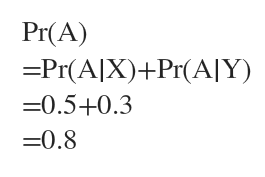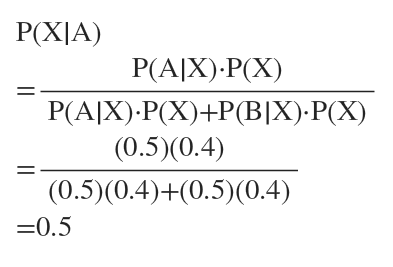# the first outcome is one of X and Y the second outcome is one of A and B if the first outcome is X, and one of A, B, and C if the first outcome is Y. Pr[X]=0.4Pr[X]=0.4Pr[Y]=0.6Pr[Y]=0.6Pr[A|X]=0.5Pr[A|X]=0.5Pr[B|X]=0.5Pr[B|X]=0.5Pr[A|Y]=0.3Pr[A|Y]=0.3Pr[B|Y]=0.2Pr[B|Y]=0.2Pr[C|Y]=0.5(1) Pr[A]=(2) Pr[X|A]=Pr[X|A]= (3) Pr[Y|B]=(4) Pr[B|Y]=

Question
18 views

the first outcome is one of X and Y the second outcome is one of A and B if the first outcome is X, and one of A, B, and C if the first outcome is Y.

Pr[X]=0.4Pr[X]=0.4
Pr[Y]=0.6Pr[Y]=0.6
Pr[A|X]=0.5Pr[A|X]=0.5
Pr[B|X]=0.5Pr[B|X]=0.5
Pr[A|Y]=0.3Pr[A|Y]=0.3
Pr[B|Y]=0.2Pr[B|Y]=0.2
Pr[C|Y]=0.5

(1) Pr[A]=

(2) Pr[X|A]=Pr[X|A]=

(3) Pr[Y|B]=

(4) Pr[B|Y]=

check_circle

Step 1

Since we only answer up to 3 sub-parts, we’ll answer the first 3. Please resubmit the question and specify the other subparts (up to 3) you’d like answered.

1) The first outcome can occur in 2 ways, X or Y.Step 2

2) Use Bay's theorem:...

### Want to see the full answer?

See Solution

#### Want to see this answer and more?

Solutions are written by subject experts who are available 24/7. Questions are typically answered within 1 hour.*

See Solution
*Response times may vary by subject and question.
Tagged in